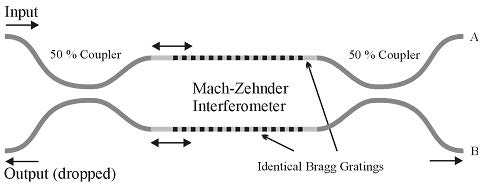In this category, we shall study the devices, where a grating is present, BPM is unsuitable (see Figure 5). Of course, as it was mentioned we can use CMT, i.e. the OptiGrating product. At this point, we may recognize the final item of our new method. To analyze some advanced structures by means of several independent techniques, we will have to connect all the particular results using some common way. Moreover, we will need a common environment to do so.

Concerning the functionality of the circuit, two identical Bragg gratings are designed to have the reflection maximum, say, at λ0   =1550nm . For example, the principal parameters of the sine groove grating are as follows: = 5mm , Δ n = 0.0007 .Figure 5: Mach-Zehnder interferometer

The device functionality is quite simple. It is the well-known Mach-Zehnder interferometer, where the arms contain an identical Bragg grating. If the wavelength of the light launched into the input port is far from the grating resonant wavelength λ0 , the light does not “see” any grating and the light thus recombines at the output coupler and emerges in the lower output port (called “B” in the following). However, when the launched light has the wavelength close to λ0   = 1550nm , a portion (the reflection should be 100% at λ0  in the case of an ideal Bragg grating) of the light is reflected by each arm and then recombines at the first/input coupler. The light is thus dropped in the lower left (“input”) port.